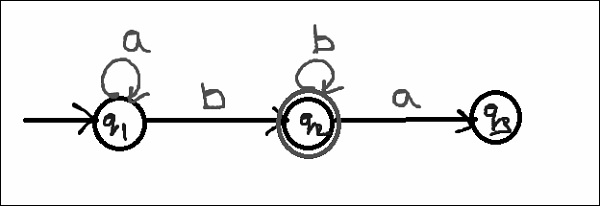# Find out the Regular expression for the given finite automata

## Problem

Construct a regular expression for the given finite automata.## Solution

Step 1 − Let us write down the equation.

q1=q1a+ ε

q1 is the start state and ε will be added as the input a which is coming to q1 from q1.

Hence, we write

State=source state of input * input coming to it

Step 2 − Similarly,

q2=q1b+q2b

q3=q2a

Step 3 − Let us simplify q1 first.

q1=q1a+ ε

we can re-write it as follows−

q1= ε +q1a

Which is similar to R=Q+RP which has a solution as follows−

R=QP*

Step 4 − Assuming R=q1, Q= ε, P=a

We get q1 = ε.a* since ε.R=R

q1=a*

Step 5 − Substituting values of q1 in q2 we get,

q2= q1a+q2b

=a*b+q2b

Step 6 − We compare this equation with R=Q+RP

Assuming R=q2, Q=a*b, P=b

We get, q2=a*b.b* as RR*=R+

We will get q2=a*.b+

Step 7 − From the given finite automata, if we want to find out the regular expression, we normally calculate the equation for the final state.

Since in the given finite automata, q2 is a final state and q2=a*b+